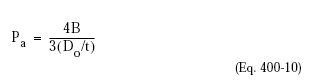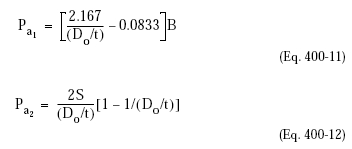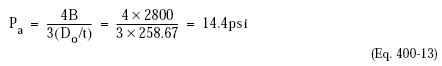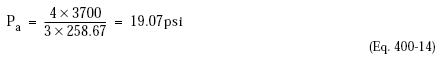﻿ Cylindrical Shell Under External Pressure | Oil & Gas Industry Technology Updates

## Cylindrical Shell Under External Pressure

Per Code Paragraph UG-28(f), vessels intended for service under external working pressures of 15 psi or less and which are to be stamped with the Code symbol are designed for a maximum allowable external pressure of 15 psi, or 25% more than the maximum possible external pressure, whichever is smaller.

The ASME procedure for cylinders under external pressure is described in detail in Paragraph UG-28. It has two different alternatives, for ratios Do/t >= 10 and Do/t <10. where: Do = External diameter of the vessel t = Minimum required thickness of the cylindrical shell L = Design length of a vessel section determined as indicated in Paragraph UG-28(b) Usually, the thickness is first determined from the internal pressure then the external pressure calculation is used to determine if this thickness is adequate for the external design pressure, or if additional stiffening of the shell is required. If the thickness of the shell has to be determined to start the procedure, a value for t must be assumed. The Code procedure then goes through several steps for each alternative in order to find the value of factor “B,” determined by using a general geometric chart (Figure 5—UGO-28.0 in Appendix 5) and a material chart for maximum design metal temperature (Appendix 5). For Do/t >= 10, the maximum allowable external pressure is determined using the equation:For Do/t <10, two values, Pa1 and Pa2 should be determined and the lower value is used:where S is the lesser of two times the allowable stress in tension or 0.9 times the yield strength of the material.
Example. Using the same vessel as in the internal pressure calculation:

• Tangent to tangent length: 36 ft 0 in. = 432 in.
• Two 2:1 semiellipsoidal heads
• External design pressure: 15 psig at 500°F

L = 448 inches (length of shell plus one-third of the depth of each head, 16 in.)
L/Do = 448/97 = 4.62
Do/t = 97/0.375 (corroded) = 258.67

From geometrical chart Figure 5, UGO-28.0, A = 0.00007. From Figure 5, UCS-28.2 for SA 515-70 at 500°F, the modulus of elasticity of material, E = 27,000,000 psi; and for A = 0.00007, the value falls to the left of the applicable temperature line. Then Pa = 2AE/3 (Do/t) = 2 x 0.00007 x 27 x 106/3 x 258.67 = 4.87 psi.

The vessel is good for only 4.87 psi external pressure, so stiffening rings are required.

Try two stiffening rings equally spaced between tangent lines.
L = 144 (length of shell between rings) + 8 (1/3 depth of head)
= 152 in.
L/Po = 152/97 = 1.56
A = 0.00022 from chart.
B = 2800 from Figure 5, UCS-28.2The vessel is still not good for 15 psi, so another ring should be added. Try three stiffening rings equally spaced between tangent lines.
L = 108 + 8 = 116 in.
L/Do = 116/97 = 1.19
A = 0.00027 from chart
B = 3700 from chart Figure 5, UCS-28.2Since Pa is greater than the design pressure, the vessel with three stiffening rings is good for full vacuum (15 psi).

Categories: Mechanical and Structural | Leave a comment